American Journal of Theoretical and Applied Statistics
Volume 4, Issue 6, November 2015, Pages: 527-533

Modelling a Pay-As-You-Drive Insurance Pricing Structure Using a Generalized Linear Model: Case Study of a Company in Kiambu

Charity Mkajuma Wamwea, Benjamin Kyalo Muema, Joseph Kyalo Mung’atu

Department of Statistics and Actuarial Sciences, Jomo Kenyatta University of Agriculture and Technology, Nairobi, Kenya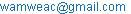(C. M. Wamwea)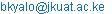(B. K. Muema)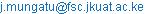(J. Mung’atu)

Charity Mkajuma Wamwea, Benjamin Kyalo Muema, Joseph Kyalo Mung’atu. Modelling a Pay-As-You-Drive Insurance Pricing Structure Using a Generalized Linear Model: Case Study of a Company in Kiambu.American Journal of Theoretical and Applied Statistics.Vol.4, No. 6, 2015, pp. 527-533. doi: 10.11648/j.ajtas.20150406.23

Abstract: The current fixed car-year pricing of auto insurance is inefficient and actuarially inaccurate since motorists in the same risk class pay the same amount of premium regardless of the number of miles covered by the different vehicles. In this paper, a simple alternative, the pay as you drive insurance, was proposed whereby motorists only pay for the mileage covered by their vehicles. The main objective was to find a suitable probability distribution that would be used to model the per kilometer risk premiums for the total aggregate claims cost. A case study was done for a company in Kiambu county. The data collected consisted of 5 variables in 194 categories whereby the total aggregate claims cost was the dependent variable. The data collection technique was via a census. The most appropriate model was found to be the zero inflated negative binomial model. The significant factors were found to be the make of the vehicle, annual mileage, and present value of the vehicle. In addition to this, mileage was also found to be positively correlated to the total aggregate claims cost.

Keywords: Pay As You Drive, Generalized Linear Model, Risk Premium, Vehicle Insurance, Total Claims Cost, Correlation, Premium Pricing

1. Introduction

 In their paper stated that the current automobile pricing models are too generalized and hence not adequate to capture the uniqueness of their individual users. This is because its pricing structure does not include relevant parameters such as mileage, driving behavior, location and the type of roads the vehicle is driven in. However, under the pay as you drive (PAYD) automobile option; these parameters are factored in converting the insurance pricing structure from fixed to variable cost . The vehicle owners, under this structure, pay premiums according to the usage of their vehicles. With this incentive, most motorists tend to reduce their mileage in the hope of paying lower premiums and as a result, this has led to a reduction of about 3% in claim frequency .

The implementation of PAYD insurance is still relatively new due to unfavorable regulations kept in place in the past. This has slowly changed as favorable legislations such as bills HB45 and HB3871 are being passed that encourage PAYD insurance, boosting its implementation. To boost the uptake of PAYD insurance to consumers,  suggested that the customers’ perceptions towards the usage of different rating factors should be considered. In his research, he found that the consumers preferred the use of risk factors that they understood. This was with regard on how they were applied in the premium calculation and the impacts it had on the premium amounts.

Currently, there are several insurance companies that offer PAYD insurance such as Progressive insurance, Real insurance, Hollard insurance, Oakhurst insurance to mention but a few. However, this type of product is not available in Kenya due to reasons such as fear of resistance from the Kenyan market, no set out legislation for such insurance by the insurance regulatory body and PAYD insurance still being an unknown concept to the Kenyan people. However, this is set to change with the introduction of a risk-based insurance system in Kenya.

 Suggested that PAYD pricing options can broadly be subdivided into three main categories namely: pay at the pump, distance-based and GPS-based premiums. However, in order to get actuarially accurate premiums, relevant risk factors should be used. According to , experience of the driver is negatively correlated to the frequency of claims. In addition to this, they found that the urban drivers were more prone to accidents as compared to rural drivers. Also, they found that night time driving only affected women and not men.  found that the number of claims recorded in year were negatively correlated to the age of the driver.

In this study, focus was on the per kilometer premiums. A simple way of calculating the PAYD premiums via this option was by dividing the annual premium with the average annual kilometers recorded in a risk class. The billing process required the premiums to be paid in advance for the annual mileage a policyholder expects to cover. If the expected number of miles was exhausted before the end of the term, the insured would have been required by the insurance company to purchase additional insurance for more mileage. However, according to , these premiums should decline up to a certain maximum amount and then they should stop. In addition to this, a minimum premium amount should be set that will cater for the expenses incurred when the policy was issued.

PAYD premiums use mileage data to convert the annual premium from fixed to variable cost. Therefore, credible data should be used. However, odometer fraud has been a major challenge.  suggested some ways on how the insurance companies can eliminate this problem. This could be done through regular odometer audits, occasional random spot checks, outsourcing staff who will be authorized to perform the odometer audits on their behalf or through the installation of an electronic device that could transmit mileage data automatically to the insurer’s database.

The main aim of this paper was to find a suitable probability distribution for the total aggregate claims cost using a generalized linear model (GLM). The concept of GLMs was first introduced in . However, in the recent years, the use of GLMs to model insurance data has been on the rise thanks to great publications and guides on its application on insurance data.

There are two main approaches that may be employed in the process of predicting the total aggregate claims cost. The first approach is through modeling the total aggregate claims cost directly using an appropriate probability distribution.  suggested that a tweedie model with 1<p<2 may be used to achieve this. Alternatively, the total aggregate claims cost can be done by modeling the claim frequencies and the claim severity separately and then combining them in the end. However, the evaluation of the claim frequency and the claim costs separately is considered to be more relevant since the risk factors influencing the two components of the insurance premium are usually different .

There are various researchers who used the GLM approach to find an appropriate model for predicting the claim frequencies.  compared various probability distributions the Poisson, negative binomial and quasi-Poison models. They found the negative binomial model to be the most appropriate since there was presence of over-dispersion in their data.  also wanted to find an appropriate model to predict the annual claim frequencies; they only used a Poisson regression model and found that it fitted well to their data.  compared the Poisson model to the negative binomial model. They found the negative binomial distribution to be the best model due to the presence of over dispersion in their data

 Compared different models such as the exponential, gamma, log-normal and the Weibull distributions and found that the log-normal was the most appropriate in predicting the claim severity of First Assurance data.  analyzed the third party Swedish data collected in 1977 using Poisson regression and other data mining techniques. He found that the Poisson probability distribution with a logit link to be the most appropriate of them all.

In this study, the data was tested for both over dispersion and zero inflation since in the insurance industry, the total claims data has a lot of zeroes due to no claims filed.

2. Methodology

Secondary data was collected from one of the companies located in Kiambu county, Kenya. The census technique was used as the data collection technique. Information on all the years the vehicles were in service was analyzed. The data analyzed consisted of one response variable (the total aggregate claims cost) and four explanatory variables (the make of the vehicle, annual mileage covered, engine capacity and the make of the vehicle). A generalized linear model was then used. The data was analyzed using the open source software R, version 3.1.2

2.1. Generalized Linear Models

Generalized linear models (GLMs) linearize the non-linear relationship between the linear predictor and the response variable. GLMs belong to the exponential family and hence their probability distributions can be expressed in the form,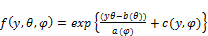(1)

where Y is the total aggregate claims cost,is the natural parameter or canonical link and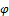is the scale or dispersion parameter. The mean and variance of the response variable used in the exponential family is given by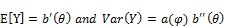(2)

GLMs consist of three major components: the random component, systematic component and the link function. The random component describes the characteristics of the response variable and assumes a probability distribution for it. The total aggregate claims cost is distributed as a compound function. The probability distributions that were used in this study were the Poisson, negative binomial, zero inflated Poisson and the zero inflated negative binomial.

The specific component specifies the predictor variables for the model. These variables enter into the model linearly. The combination of these factors is the linear predictor. The multiple linear predictor used in this study was given by:(3)

where X is a matrix that contains the explanatory variables: MAKE, VALUE, CC and KM,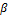is a vector consisting of the regression parameters to be estimated by the model, e is a vector consisting of the errors which is multivariate normal,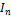is an nxn identity matrix,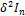is the covariance matrix.

The link function specifies a function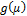relating to the linear predictor. It acts as the connector between the random component and the systematic component. The link function is given by: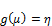(4)

where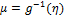is the mean of the total aggregate claims and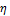is the linear predictor specified in equation (3). Table 1 consists of commonly used link functions for different distributions.

Table 1. Commonly used Link Functions.

 Distribution Link Name Link Function Normal Identity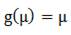Poisson Log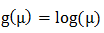Binomial Logit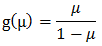Gamma Inverse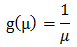The GLM parameters are estimated via the maximum likelihood estimation (MLE) technique. This is achieved when the log likelihood function given by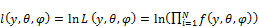(5)

is maximized so as to produce the maximum likelihood estimates. This can easily be done in R through the use of iterative procedures such as the Newton Raphson algorithm given by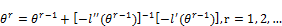(6)

where,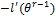and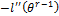are the first and second derivatives of equation (5) evaluated at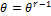, or the Fischer scoring algorithm given by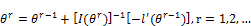(7)

where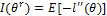is the Fischer’s information matrix.

2.2. Assessment of Goodness of Fit

Deviance is given by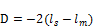(8)

where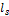is the log likelihood function of the saturated model while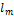is the log likelihood of the proposed model. Deviance is used to compare the fit of the proposed model to the fit of the saturated model. The value of D is then compared with the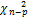where n is the number of observations and p is the number of parameters. The proposed model is assumed to be unsuitable whenat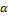level of significance.

Alternatively, the generalized chi-square goodness of fit test given by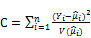(9)

where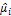and V(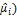are respectively the estimated mean and variance. The proposed model is also assumed to be a lack of good fit when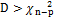at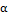level of significance.

There is a need of knowing the number of appropriate parameters to be included in the model and still obtain a good fit. An assessment of the significance of the explanatory variables is done. The Wald test given by(10)

where SE is the standard error and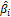is the value of the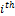estimated parameter. Z is compared with the standard normal distribution. The explanatory variable is considered to be insignificant when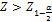atlevel of significance.

2.4. Model Selection

Once the assessment of goodness of fit is done, good models are found. Therefore, there is need to pick the finest model amongst them. This can be achieved through the use of the information criterions such as the Akaike Information Criterion (AIC) and the Bayesian Information Criterion (BIC) given by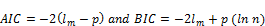(11)

whereis the log likelihood of the proposed model and p is the number of parameters in the proposed model. The preferred model is the one with the smallest AIC or BIC.

2.5. Test for Over-Dispersion

Given the variance function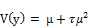(12)

whereis the over dispersion parameter and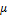is the mean of Y, the total aggregate claims cost. According to , the test statistic for over-dispersion is given by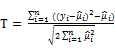(13)

and Y is considered to be over-dispersed when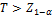whereis the level of significance and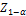can be found in the standard normal tables.

2.6. Vuong Closeness Test

Vuong  came up with a likelihood test based on the Kull Leibner information criteria. It tests whether the two models, the simpler and the complex one, are close to the true specification against the alternative that the complex model is closer to the true specification. The test is given by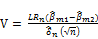(14)

where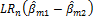is the summed difference between the log likelihoods of the two models given by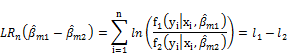n is the number of observations and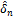is the estimated standard deviance given by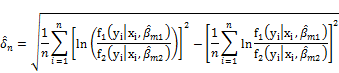However, the vuong test is affected by the number of estimated parameters and hence the test needs to be corrected for model dimensionality. A correction to the vuong statistic related to the AIC or BIC is used to solve the problem. The adjusted vuong test statistic is now given by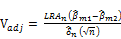(15)

whereand p and q are respectively the number of parameters in models 1 and 2. The complex model is considered to be closer to the true specification whenor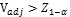atlevel of significance.

3. Results and Discussion

This section presents the results obtained through the application of the methodology discussed in section 2. The discussion was then based on the findings. The level of significance used throughout this study was at 5%.

3.1. Dataset Description

Data collected on five variables: annual mileage, make of the vehicle, engine capacity, present value of the car and the total aggregate claims cost was analyzed and used in the calculation of the per kilometer risk premiums.

3.1.1. Total Aggregate Claims Cost

This was the response variable. It contained the total aggregate amount of money claimed by a vehicle per year in Kenyan shillings. Its descriptive statistics were displayed in Table 2.

Table 2. Descriptive Statistics of the Total Aggregate Claims cost.

 Min Mean Mode Median Std.dev Max 0 5151 0 0 9085 48500

Table 2 shows that the mean, median and mode are not equal. In addition to this, it showed that most of the total aggregate claims amounts were zero. Some of the reasons why this was so were: 1) many of the vehicles were not involved in an accident, 2) some of those that were, recorded claims that did not exceed the deductible amount, and 3) some of the claims were not reported to the insurance company as they were considered too small. A histogram of the total aggregate claims cost was plotted so as to see how it is skewed.Figure 1. Histogram the Total Aggregate Claims cost.

The histogram in Figure 1 demonstrated that the data was positively skewed to the right. This implied that the use of a Gaussian model could have been inappropriate and hence a Shapiro-wilk test for normality was done on the data to ascertain this. The null hypothesis being that the data was normally distributed against the alternative that it was not. The results were then displayed in Table 3.

Table 3. Results from the Shapiro-Wilk test.

 Statistic P-value 0.6383 <2.2e-16

From these results, it was found that the data was in deed not normally distributed at 5% level of significance.

3.1.2. Mileage

This variable consisted of the annual mileage a vehicle has covered over the year. The overall annual mileage was found to be 27,685 kilometers. The mileage data was then classified as in Table 4.

Table 4. Classification of Annual Mileage.

 Classification Interval Frequency 1 0-1000 1 2 1001-10000 25 3 10001-30000 91 4 30001-47000 49 5 >47000 28

3.1.3. Engine Capacity

The engine capacity ranged between 100 and 13,741 cc. The values of the different engine capacities were then classified as in Table 5.

Table 5. Classification of Vehicle Engine Capacity.

 Classification Interval Frequency 1 0-2000 80 2 2001-3000 63 3 >3000 51

It was seen that most vehicles had an engine capacity ranging from 0 to 2000 cc.

3.1.4. Make of the Vehicle

There were several car models considered in this study and they were classified as in Table 6 due to their frequencies.

Table 6. Classification of the Make of the vehicle.

 Classification Interval Frequency 1 Isuzu 48 2 Mitsubishi 34 3 Toyota 101 4 Others 11

This shows that most of the vehicles in this study were Toyota branded followed by Isuzu then Mitsubishi.

3.1.5. Present Value of the Vehicle

The present values of the vehicles were recorded and categorized in Table 7.

Table 7. Classification of the Present Value of the Vehicle.

 Classification Interval Frequency 1 0-1000000 28 2 1000001-25000000 129 3 >2500000 37

3.2. Finding a Suitable Distribution for the Total Aggregate Claims Cost

The first assumption was that the total aggregate cost followed a compound Poisson distribution and hence a Poisson distribution was fitted to the data. However, there are times that the data shows the presence of over-dispersion. Hence, if this was the case, it would be inappropriate to model the data via a compound Poisson model. A test for over dispersion, discussed under section 2.4, was done and the results were as recorded in Table 8.

Table 8. Results from the over dispersion test.

 T Statistic P-Value 2.5678 0.005117

From the results in Table 8, it was found that the data was over-dispersed. This implied that it was necessary to fit another distribution to the data that catered for the over-dispersion in the data. Therefore, a negative binomial model was fitted to the data.

In addition to this, it was seen from the total aggregate claims cost data that there were so many zero claims recorded. Hence, there was need to perform a zero inflation test on the total aggregate claims cost. Therefore, two more distributions were fitted to the data: the zero inflated Poisson model and the zero inflated negative binomial model. Two vuong tests were performed on the data; one of them between the Poisson and the zero inflation Poisson model and the other between the negative binomial and the zero inflated negative binomial model. The results from the vuong tests were as recorded in Table 9 and 10 respectively.

Table 9. Vuong Test between Poisson and ZIP.

 Type Vuong Statistic p-value Raw -4.681712 1.4224e-06 AIC-corrected -4.674503 1.4733e-06 BIC-corrected -4.669001 1.5133e-06

From the results in Table 9, it was found that the zero inflated Poisson (ZIP) model was closer to the true specification compared to the Poisson model.

Table 10. Vuong Test between NB and ZINB.

 Type Vuong Statistic p-value Raw -10.006578 <2.22e-16 AIC-corrected -9.124027 <2.22e-16 BIC-corrected -7.682002 7.311e-15

Also, from the results in Table 10, it was found that the zero inflated negative binomial (ZINB) model was closer to the true specification compared to the negative binomial (NB) model. Hence, the two zero inflated models were found to be better fitting models compared to their counterparts implying that zero inflation was present in the data.

The AIC and log-likelihood values of the fitted models were recorded in Table 11.

Table 11. AIC and the Log-Likelihood values of the Fitted Models.

 Model AIC Log-Likelihood Pois 1,515,921.00 -756,949.70 NB 1,865.70 -920.87 ZIP 208,260.10 -104,106.00 ZINB 1,596.95 -773.48

Table 12. Results on the Stepwise Regression on ZINB Model.

 Variable Df AIC -factor(cc) 4 1596.7 none 1597.0 -factor(km) 8 1607.7 -factor(val) 4 1655.1 -factor(Mk) 6 1636.7

The results from Table 11 show that the zero inflated negative binomial model had the smallest AIC making it the most appropriate model out of the four. This was because the data was both zero inflated and over-dispersed.

Stepwise regression was performed on the fitted negative binomial model so as to get the best combination of factors that would yield the lowest AIC. The results from the stepwise regression were recorded in Table 12.

From the results on the stepwise regression in Table 12, it was seen that when all the four explanatory variables were fitted together in the data, the AIC was at 1597.0. However, when the variables make, value and make were eliminated, the AIC went up. However, when the engine capacity factor was eliminated, the AIC value went down to 1596.7. This implied that the engine capacity factor was not relevant in predicting the total claims cost distribution. However, the make of the vehicle, annual mileage and the present value of the vehicle were found to be significant to the study.

Another zero inflated negative binomial model was fitted to the data with only the three significant factors. The estimated parameters were displayed as in Table 13.

Table 13. Estimated Parameters from the Best fitted ZINB Model.

 Variable Df AIC Intercept 7.86475 -16.53201 factor(Mk)2 -0.06608 -0.32200 factor(Mk)3 0.13310 2.15850 factor(Mk)4 1.29349 0.09608 factor(km)2 0.74332 15.75723 factor(km)3 0.94231 14.11106 factor(km)4 1.02109 13.84011 factor(km)5 1.54756 13.84683 factor(val)2 0.66832 1.96595 factor(val)3 0.05960 4.93554

3.3. Determining the Effect of Mileage as a Risk Factor

A two-sided test for correlation was performed on the data so as to test whether the true correlation,, between mileage and the total aggregate claims cost was equal to zero against the alternative that it was not. The results from the test were as recorded in Table 14.

Table 14. Two-sided Pearson Product Moment Correlation Test.

 Statistic P-value Alternative 2.9659 0.0042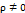From the results displayed in Table 14, it was found that the true correlation between mileage and total aggregate claims cost was significantly not equal to zero. Therefore, two more one-sided Pearson product moment correlation tests were performed on the two variables. The results were recorded in Table 15.

Table 15. One-sided Pearson Product Moment Correlation Tests.

 Statistic P-value Alternative 2.9659 0.9979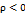2.9659 0.0021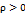From the results in Table 15, it was found that there was positive correlation between mileage and the total aggregate claims cost implying that the total aggregate claims cost increased with every mileage increase.

4. Conclusions and Recommendations

This section presents the conclusions derived from the results and the recommendations made for further research.

4.1. Conclusions

One of the aims of this research was to find an appropriate model for the total aggregate claims cost and it was found to be the zero inflated negative binomial model. The make of the vehicle, annual mileage, and the present value of the vehicle were the only significant explanatory variable. In addition to this, mileage was found to be positively correlated to the total aggregate claims cost justifying why PAYD insurance should be used instead of fixed car year pricing. However, due to time restrictions, the study was constrained to a specific company located in Kiambu County. This implied that the findings in this research could not be generalized to all the institutions in Kenya but could be used as a basis for future research purposes on PAYD insurance.

4.2. Recommendations

The researcher recommends that an extension of this research should be extended to sample surveys whereby more institutions from different parts of the country are sampled so as to achieve more generalized results. In addition to this, more data will be collected making the results more reliable. Other distributions should be used so as to see whether a more appropriate model could be found.

Abbreviations

PAYD - Pay As You Drive

ln – Natural Logarithm

NB – Negative Binomial

ZINB – Zero Inflated Negative Binomial

ZIP – Zero Inflated Poisson

References

1. A. Nandeshwar, "Studying Auto Insurance Data," unpublished, 2010.
2. D. Deng and S. Paul, "Score Tests for Zero-Inflation and Over-dispersion in Generalized Linear Models," Statistica Sinica, pp. 257-276, 2005.
3. E. Ohlsson, and B. Johansson, "Non-Life Insurance Pricing with Generalized Linear Models," Springer-Verlag, Berlin, 2015.
4. H. Lennon, "Generalized Linear Models and their Extensions for Insurance Data," unpublished, 2011.
5. J. Bordoff and P. Noel, "The Impact of Pay As You Drive Auto Insurance in California," Brookings Institution, 2008.
6. J. Ferreira and E. Minikel, "Pay-As-You-Drive Auto Insurance in Massachusetts: A Risk Assessment and Report on Consumer, Industry and Environmental Benefits," Saint Paul (MI): Department of Urban Studies and Planning, Massachusetts Institute of Technology, 2010.
7. J. A. Nelder and R. W. M. Wedderburn, "Generalized Linear Models," Journal of the Royal Statistical Society, A. 135, 370-384, 1972.
8. J. Boucher, A. Pérez-Marín and M. Santolino, "Pay-As-You-Drive Insurance: The Effect of the Kilometers on the Risk of Accident," Anales del Instituto de Acturios Espanioles, 3ª Época, 19, 135-154, 2013.
9. M. A. Oyugi, Actuarial modelingfor insurance claim severity in motor comprehensive policy using industrial statistical distributions, International Congress of Actuaries, Capetown, 2010.
10. M. Ayuso, M. Guillén and A. M. Pérez-Marín, "ime and distance to first accident and driving patterns of young drivers with pay-as-you-drive insurance," Accident Analysis and Prevention, 125-131, 2014.
11. M. David, and D. V. Jemna, "Modeling the Frequency of Auto Insurance Claims by Means of Poisson and Negative Binomial Models", Annals of the Alexandru Ioan Cuza University-Economics, 62(2), 151-168, 2015.
12. M. David, "Automobile insurance pricing with Generalized Linear Models," Proceedings in GV-Global Virtual Conference, 2015.
13. S. Husnjak, D. Peraković, I. Forenbacher, & M. Mumdziev, "Telematics System in Usage Based Motor Insurance," Procedia Engineering, 100, 816-825, 2015.
14. S. Kafkova, and L. Krivankova, "Generalized linear models in vehicle insurance," Acta Universitatis Agriculturae et Silviculturae Mendelianae Brunensis, 62, No. 2, 383-388, 2014.
15. T. Litman and R. Meyer, "Pay As-You-Drive Vehicle Insurance in British Columbia," Pacific Institute for Climate Solutions, University of Victoria, 2011.
16. T. Störmer, "Optimizing insurance pricing by incorporating consumers’ perceptions of risk classification." Zeitschrift für die gesamte Versicherungswissenschaft 104.1, 11-37, 2015.
17. P. Jong, G. Z. Heller, "Generalized Linear Models for Insurance Data," International Series on Actuarial Science, Cambridge University Press, 2008.
18. Q. Vuong, "Likelihood Ratio Test form Model Selection and Non-nested hypotheses," Econometrica: Journal of the Econometric s=Society, 307-333, 1989.

 Contents 1. 2. 2.1. 2.2. 2.3. 2.4. 2.5. 2.6. 3. 3.1. 3.2. 3.3. 4. 4.1. 4.2.
Article ToolsAbstractPDF(358K)